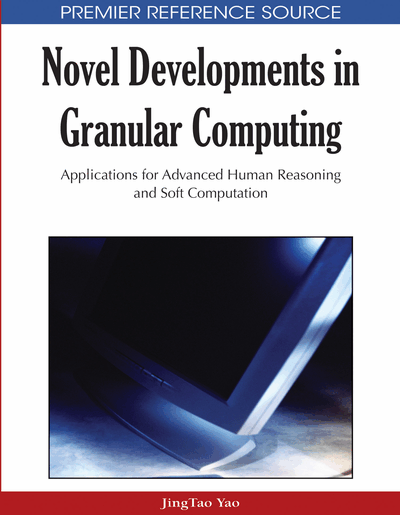# From Interval Computations to Constraint-Related Set Computations: Towards Faster Estimation of Statistics and ODEs under Interval, p-Box, and Fuzzy Uncertainty

Martine Ceberio (University of Texas at El Paso, USA), Vladik Kreinovich (University of Texas at El Paso, USA), Andrzej Pownuk (University of Texas at El Paso, USA) and Barnabás Bede (University of Texas - Pan American, USA)
DOI: 10.4018/978-1-60566-324-1.ch006
Available
\$33.75
List Price: \$37.50
10% Discount:-\$3.75
TOTAL SAVINGS: \$3.75

## Abstract

One of the important components of granular computing is interval computations. In interval computations, at each intermediate stage of the computation, we have intervals of possible values of the corresponding quantities. In our previous papers, we proposed an extension of this technique to set computations, where on each stage, in addition to intervals of possible values of the quantities, we also keep sets of possible values of pairs (triples, etc.). In this paper, we show that in several practical problems, such as estimating statistics (variance, correlation, etc.) and solutions to ordinary differential equations (ODEs) with given accuracy, this new formalism enables us to find estimates in feasible (polynomial) time.
Chapter Preview
Top

## 1. Formulation Of The Problem

### 1.1. Need for Data Processing

Computing an estimate forbased on the results of direct measurements is called data processing; data processing is the main reason why computers were invented in the first place, and data processing is still one of the main uses of computers as number crunching devices.

## Complete Chapter List

Search this Book:
Reset